# 每日四题打卡-3.25:模拟堆/哈希表/字符串哈希/DFS排列数字

### 1、模拟堆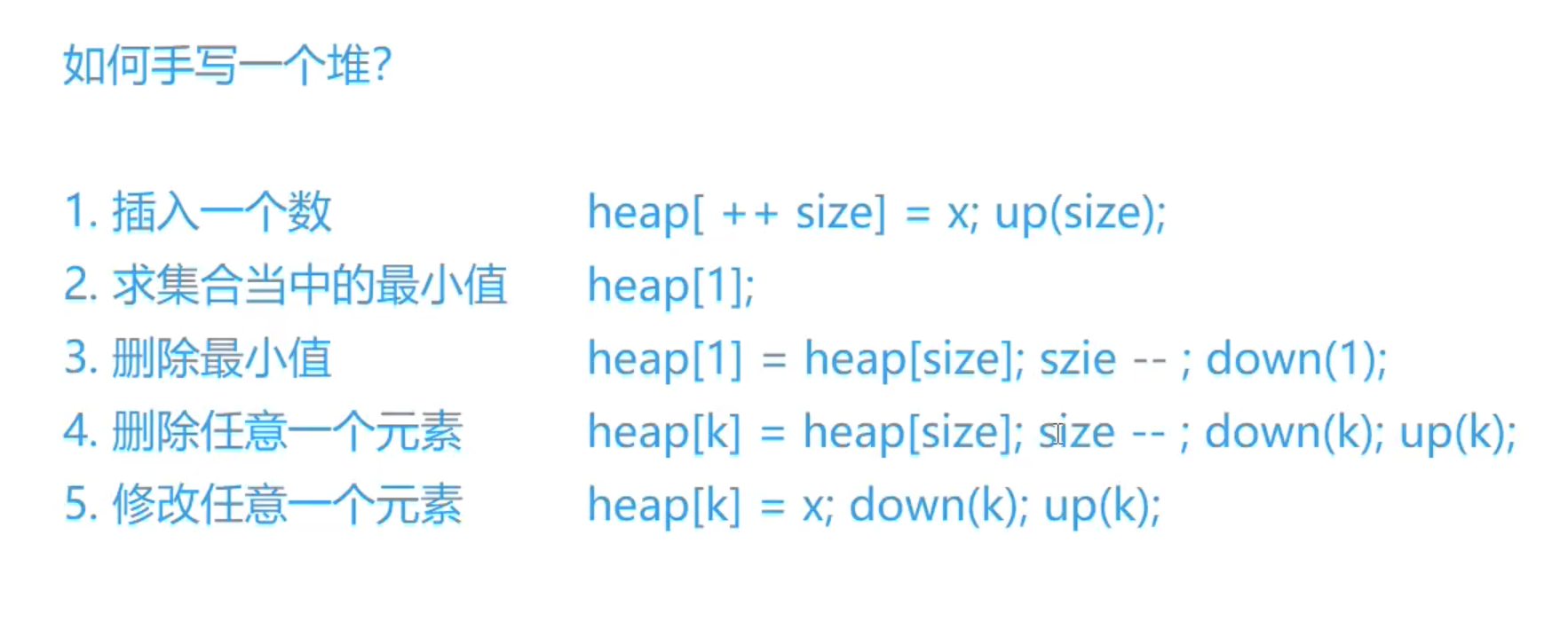1. “I x”，插入一个数x；
2. “PM”，输出当前集合中的最小值；
3. “DM”，删除当前集合中的最小值（数据保证此时的最小值唯一）；
4. “D k”，删除第k个插入的数；
5. “C k x”，修改第k个插入的数，将其变为x；

1≤N≤1051≤N≤105
−109≤x≤109−109≤x≤109

8
I -10
PM
I -10
D 1
C 2 8
I 6
PM
DM


-10
6
#include<iostream>
#include<algorithm>
using namespace std;

const int N=1e5+10;
int h[N];   //堆
int ph[N];  //存放第k个插入点的下标
int hp[N];  //存放堆中点的插入次序
int cur_size;   //size 记录的是堆当前的数据多少

void heap_swap(int u,int v)
{
swap(h[u],h[v]);
swap(hp[u],hp[v]);
swap(ph[hp[u]],ph[hp[v]]);

}

void down(int u)
{
int t=u;
if(u*2<=cur_size&&h[t]>h[u*2]) t=u*2;
if(u*2+1<=cur_size&&h[t]>h[u*2+1])  t=u*2+1;
if(u!=t)
{
heap_swap(u,t);
down(t);
}
}
void up(int u)
{
if(u/2>0&&h[u]<h[u/2])
{
heap_swap(u,u/2);
up(u>>1);
}
}

int main()
{
int n;
cin>>n;
int m=0;      //m用来记录插入的数的个数
//注意m的意义与cur_size是不同的 cur_size是记录堆中当前数据的多少
//对应上文 m即是hp中应该存的值
while(n--)
{
string op;
int k,x;
cin>>op;
if(op=="I")
{
cin>>x;
m++;
h[++cur_size]=x;
ph[m]=cur_size;
hp[cur_size]=m;
//down(size);
up(cur_size);
}
else if(op=="PM")    cout<<h<<endl;
else if(op=="DM")
{
heap_swap(1,cur_size);
cur_size--;
down(1);
}
else if(op=="D")
{
cin>>k;
int u=ph[k];                //这里一定要用u=ph[k]保存第k个插入点的下标
heap_swap(u,cur_size);          //因为在此处heap_swap操作后ph[k]的值已经发生
cur_size--;                    //如果在up,down操作中仍然使用ph[k]作为参数就会发生错误
up(u);
down(u);
}
else if(op=="C")
{
cin>>k>>x;
h[ph[k]]=x;                 //此处由于未涉及heap_swap操作且下面的up、down操作只会发生一个所以
down(ph[k]);                //所以可直接传入ph[k]作为参数
up(ph[k]);
}

}
return 0;
}

### 2、哈希表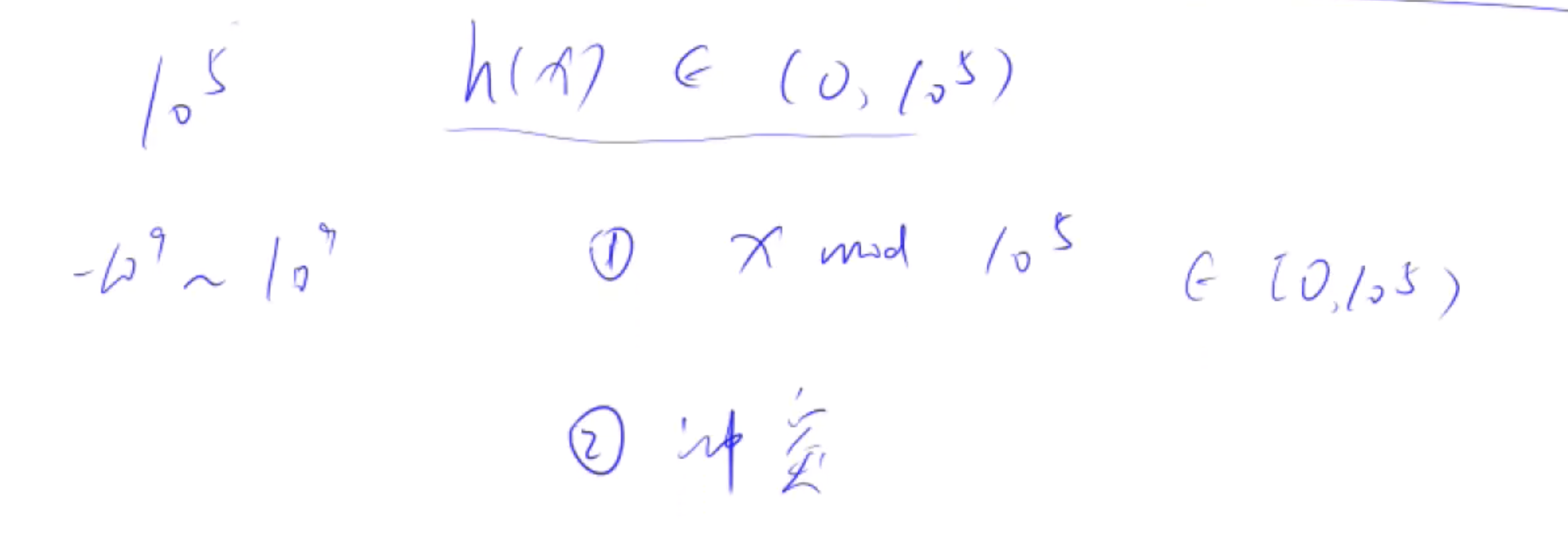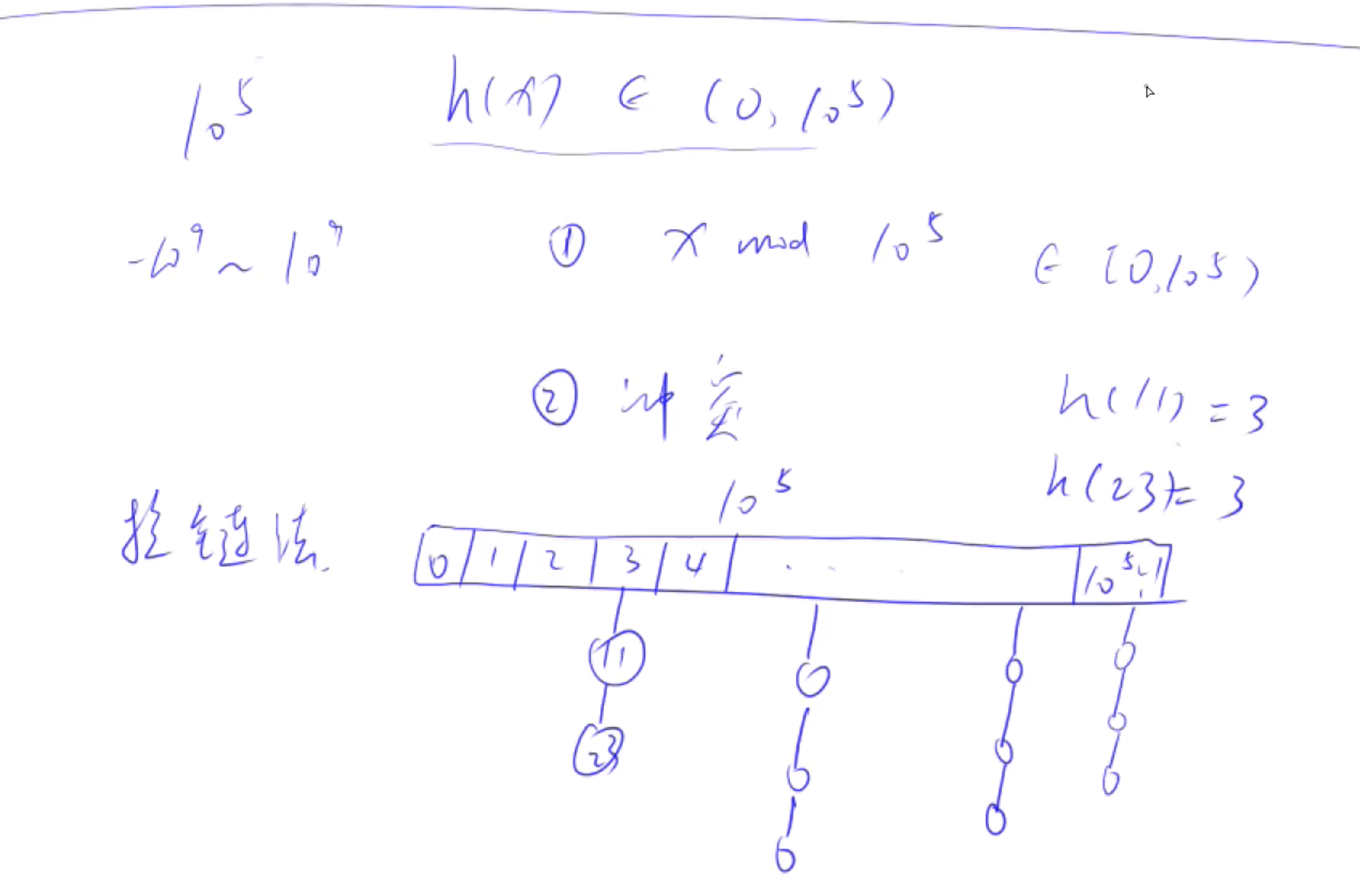1. “I x”，插入一个数x；
2. “Q x”，询问数x是否在集合中出现过；

1≤N≤1051≤N≤105
−109≤x≤109−109≤x≤109

5
I 1
I 2
I 3
Q 2
Q 5


Yes
No
//步骤：哈希表的拉链法得定义h[N]作为链表头节点，每个节点下面挂着一条链表，所以得定义链表基本的e[N],ne[N],idx
//插入操作：先把数据mod N,压缩到N以内，然后链表的插入操作：存x,ne[idx]指向第k个头节点h[k],h[k] ++;
//查找操作：先把数据mod N,压缩到N以内，然后链表的查询操作：for循环，找到一个e[i] == x的点return true;否则return false
#include <iostream>
#include <cstring>
using namespace std;
const int N = 100003;////输入的数必须是质数，100000之后的第一个质数是100003
int h[N], e[N], ne[N], idx;//h是槽，加的链是链表包括e, ne, idx

void insert(int x){
int k = (x % N + N) % N;////防止x % N < 0,保证k是正数
//链表插入操作
e[idx] = x;
ne[idx] = h[k];
h[k] = idx ++;
}

//链表查询
bool find(int x){
int k = (x % N + N) % N;
for (int i = h[k]; i != -1; i = ne[i])
if (e[i] == x) return true;
return false;
}

int main()
{
int n;
cin >> n;
memset(h, -1, sizeof h);
while (n --){
char op;
int x;
cin >> op;
cin >> x;
if (op == 'I') insert(x);
else {
if (find(x)) puts("Yes");
else puts("No");
}
}
return 0;
}


### 3、字符串哈希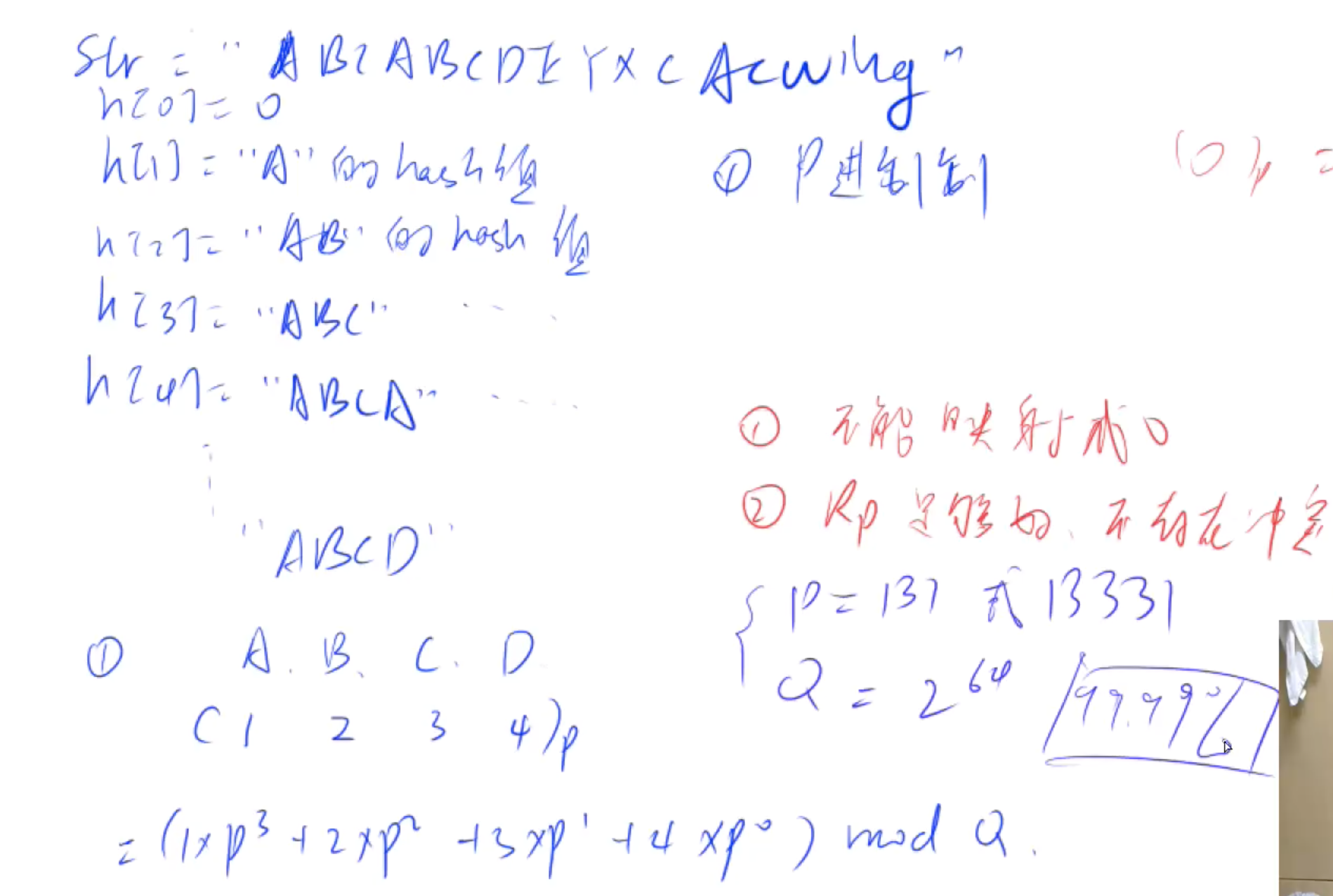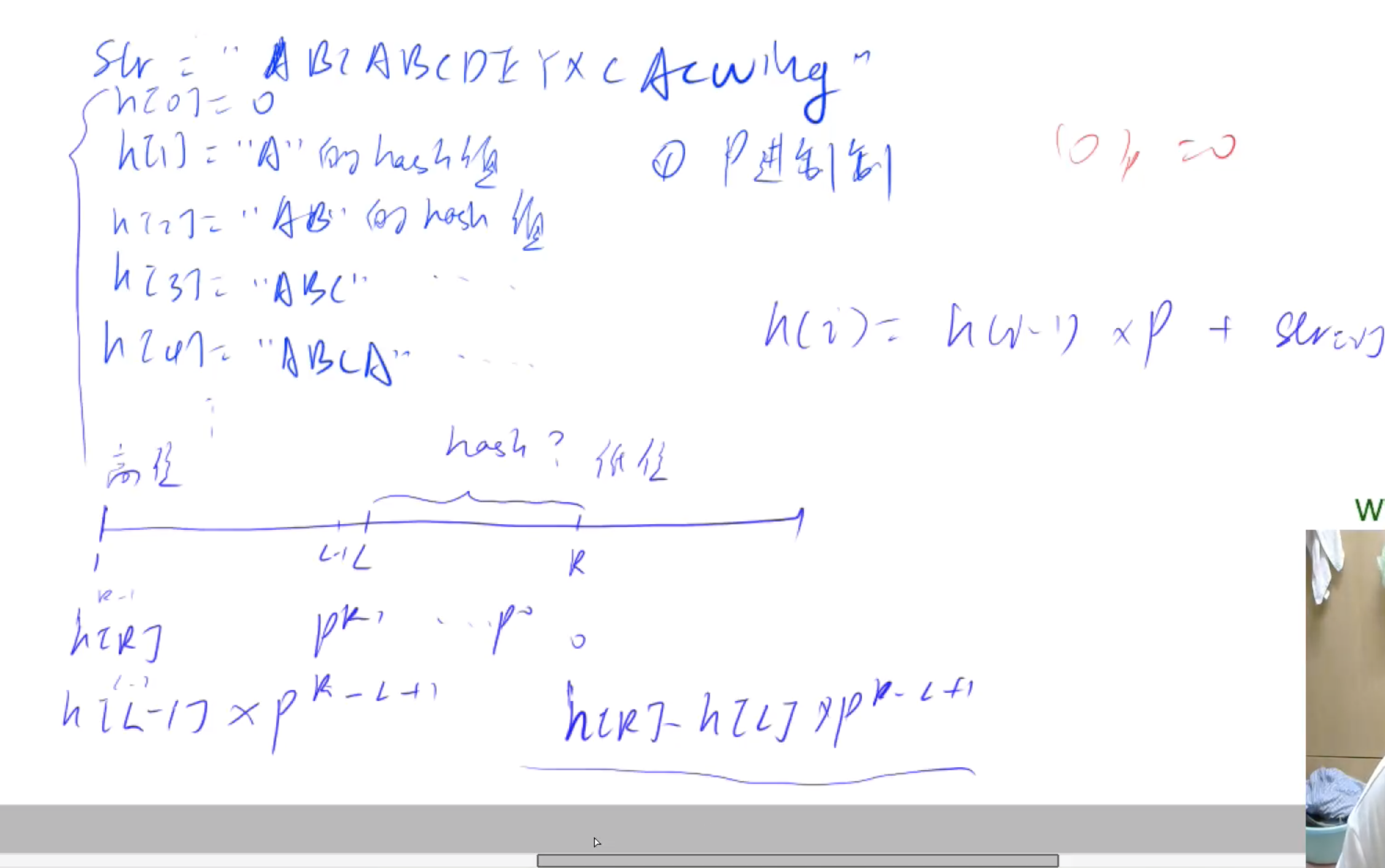1≤n,m≤1051≤n,m≤105

8 3
aabbaabb
1 3 5 7
1 3 6 8
1 2 1 2


Yes
No
Yes
//步骤：数据范围比较大所以用ULL来表示该类型变量，P是131包括字母和数字总和大小
//公式：求L到R区间的哈希值：h[r] - h[l - 1] * p[r - l + 1];
#include <iostream>
using namespace std;
typedef unsigned long long ULL;//用ULL来表示该类型变量
const int N = 100010, P = 131;
int n, m;
char str[N];
ULL h[N], p[N];//h[N]存哈希值，p[N]存P的多少次方

ULL get(int l, int r){
return h[r] - h[l - 1] * p[r - l + 1];
}

int main()
{
cin >> n >> m >> str + 1;
p = 1;
//初始化
for (int i = 1; i <= n; i ++){
p[i] = p[i - 1] * P;
h[i] = h[i - 1] * P + str[i];
}
while (m --){
int l1, r1, l2, r2;
cin >> l1 >> r1 >> l2 >> r2;
if (get(l1, r1) == get(l2, r2)) puts("Yes");
else puts("No");
}
return 0;
}


### 4、DFS-排列数字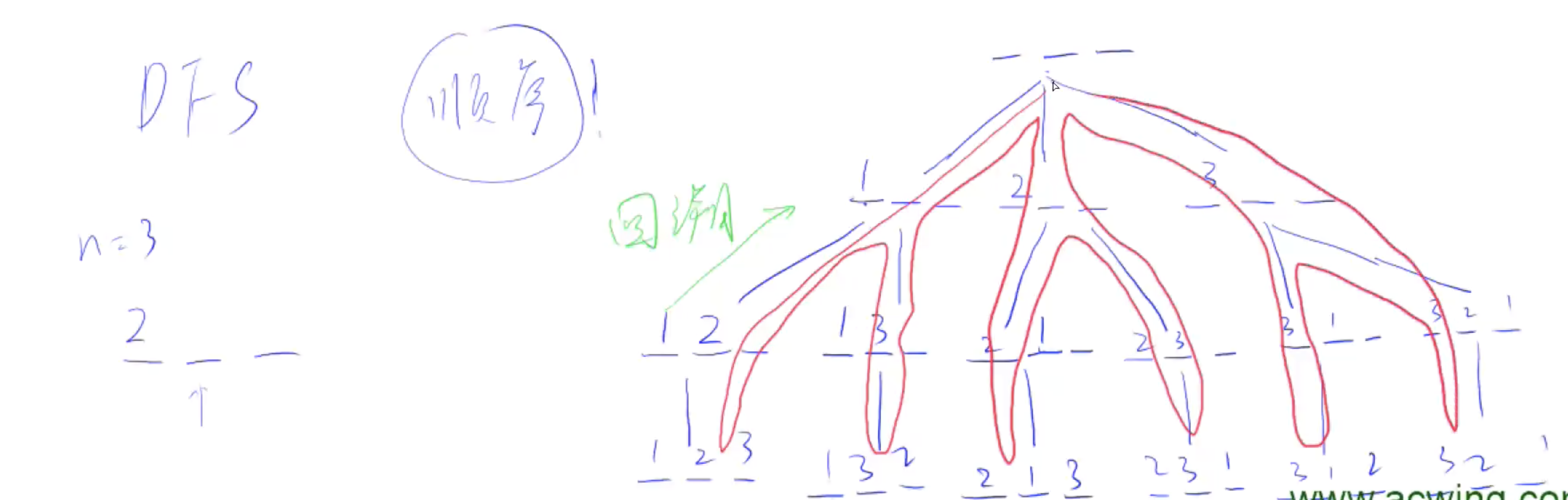1≤n≤71≤n≤7

3


//dfs步骤：
//1、当走到第n个位置，说明我们把所有位置填满了
//2、否则枚举当前位置能填哪些数，如果该数没被用过将i放到当前位置并标记，递归到下一层，最好恢复现场。
#include <iostream>
using namespace std;
const int N = 10;//数范围1-7
int n;
int path[N];//存状态
bool st[N];//当前位置需要填哪些数，需要开bool数组，如果该点为true则表示该点被用过了

void dfs(int u){
if (u == n){
//输出结果
for (int i = 0; i < n; i ++) cout << path[i] << ' ';
puts("");
return;
}
for (int i = 1; i <= n; i ++){//枚举当前位置能填哪些数
if (!st[i]){
path[u] = i;//将i放到当前位置
st[i] = true;//记录i已被用过
dfs(u + 1);//递归到下一层
//当dfs结束之后，一条遍历完所以需要恢复现场
st[i] = false;
}
}
}

int main()
{
cin >> n;
dfs(0);
return 0;
}©️2019 CSDN 皮肤主题: 编程工作室 设计师: CSDN官方博客